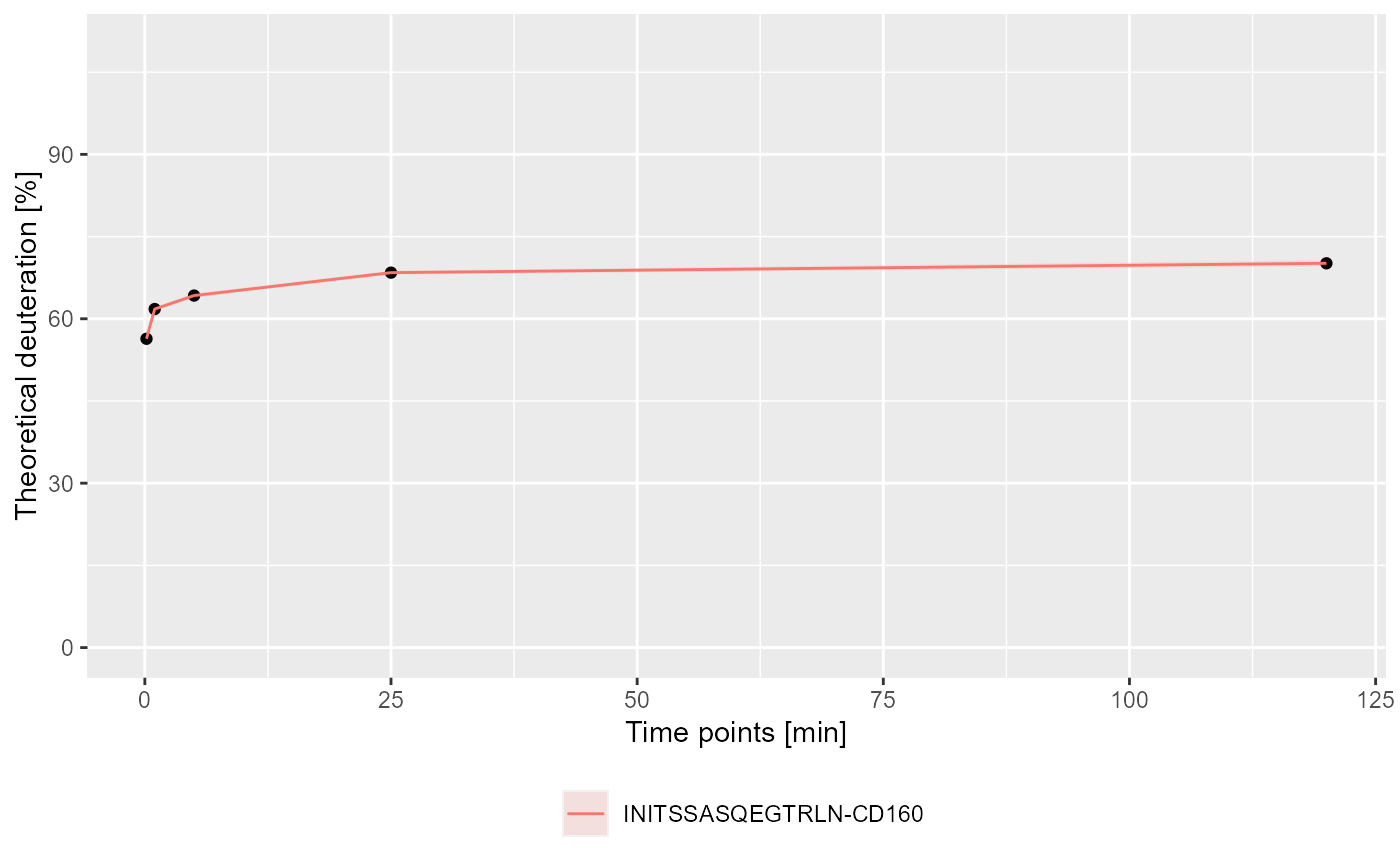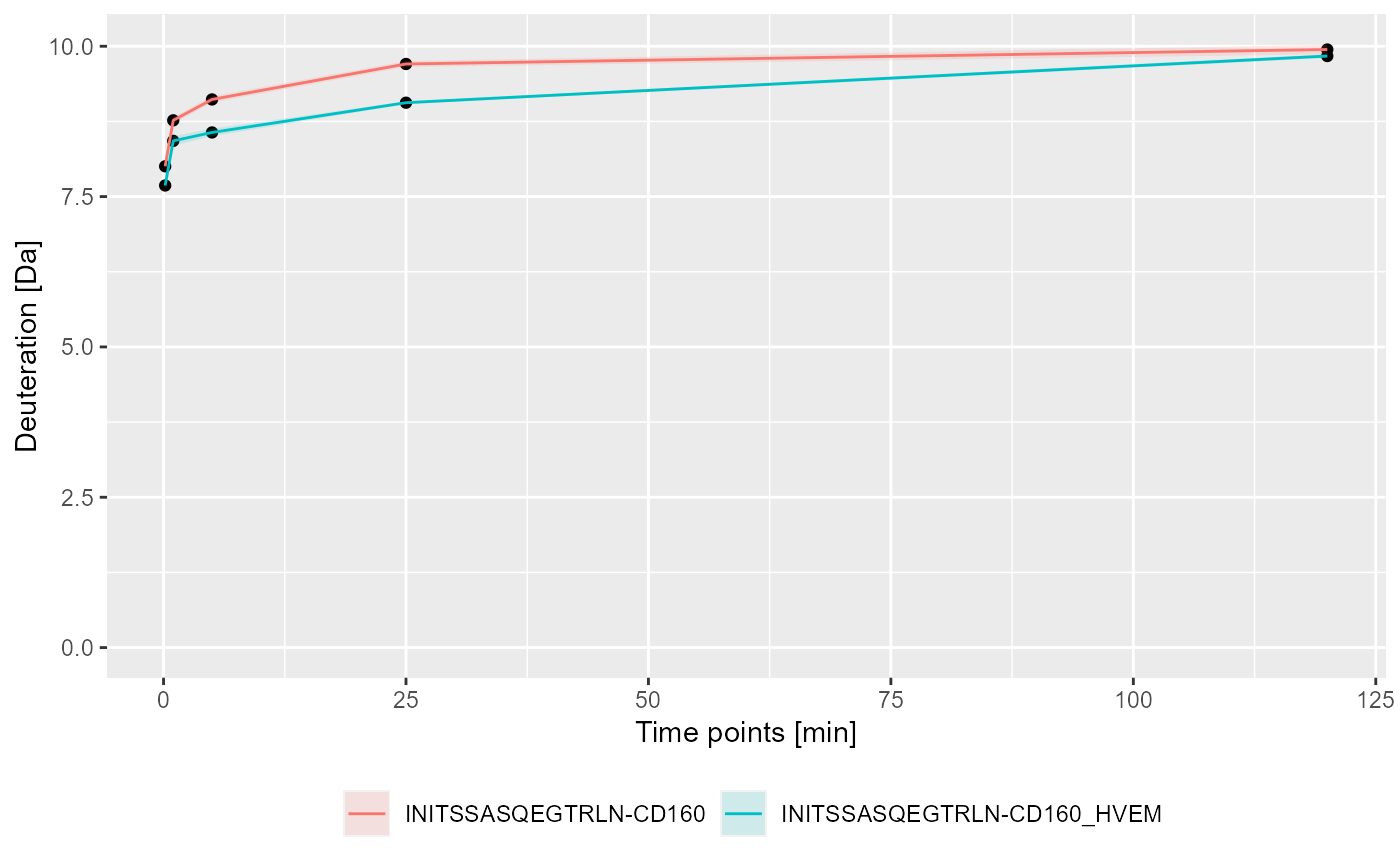Plots kinetics of the hydrogen-deuterium exchange for specific peptides.

plot_kinetics(kin_dat, theoretical = FALSE, relative = TRUE)

## Arguments

kin_dat calculated kinetic data by calculate_kinetics function logical, determines if plot shows theoretical values logical, determines if values are relative or absolute

## Value

a ggplot object.

## Details

This function visualises the output of the calculate_kinetics function. Based on supplied parameters appropriate columns are chosen for the plot. The uncertainty associated with each peptide is shown as a ribbon. Axis are labeled according to the supplied parameters but no title is provided.

If you want to plot data for more then one peptide in one state, join calculated data by using bind_rows from dplyr package and pass the result as kin_dat.

calculate_kinetics

## Examples

# load example data

# calculate data for the sequence INITSSASQEGTRLN in the CD160 state
(kin1 <- calculate_kinetics(dat,
protein = "db_CD160",
sequence = "INITSSASQEGTRLN",
state = "CD160",
start = 1,
end = 15,
time_in = 0.001,
time_out = 1440))
#> # A tibble: 5 x 15
#>   Protein Sequence Start   End State time_chosen frac_exch_state
#>   <chr>   <chr>    <int> <int> <chr>       <dbl>           <dbl>
#> 1 db_CD1~ INITSSA~     1    15 CD160       0.167            76.9
#> 2 db_CD1~ INITSSA~     1    15 CD160       1                84.2
#> 3 db_CD1~ INITSSA~     1    15 CD160       5                87.5
#> 4 db_CD1~ INITSSA~     1    15 CD160      25                93.2
#> 5 db_CD1~ INITSSA~     1    15 CD160     120                95.5
#> # ... with 8 more variables: err_frac_exch_state <dbl>,
#> #   abs_frac_exch_state <dbl>, err_abs_frac_exch_state <dbl>,
#> #   avg_theo_in_time <dbl>, err_avg_theo_in_time <dbl>,
#> #   abs_avg_theo_in_time <dbl>, err_abs_avg_theo_in_time <dbl>,
#> #   Med_Sequence <dbl>
# calculate data for the sequence INITSSASQEGTRLN in the CD160_HVEM state
(kin2 <- calculate_kinetics(dat,
protein = "db_CD160",
sequence = "INITSSASQEGTRLN",
state = "CD160_HVEM",
start = 1,
end = 15,
time_in = 0.001,
time_out = 1440))
#> # A tibble: 5 x 15
#>   Protein Sequence Start   End State time_chosen frac_exch_state
#>   <chr>   <chr>    <int> <int> <chr>       <dbl>           <dbl>
#> 1 db_CD1~ INITSSA~     1    15 CD16~       0.167            74.4
#> 2 db_CD1~ INITSSA~     1    15 CD16~       1                81.5
#> 3 db_CD1~ INITSSA~     1    15 CD16~       5                82.9
#> 4 db_CD1~ INITSSA~     1    15 CD16~      25                87.7
#> 5 db_CD1~ INITSSA~     1    15 CD16~     120                95.2
#> # ... with 8 more variables: err_frac_exch_state <dbl>,
#> #   abs_frac_exch_state <dbl>, err_abs_frac_exch_state <dbl>,
#> #   avg_theo_in_time <dbl>, err_avg_theo_in_time <dbl>,
#> #   abs_avg_theo_in_time <dbl>, err_abs_avg_theo_in_time <dbl>,
#> #   Med_Sequence <dbl>